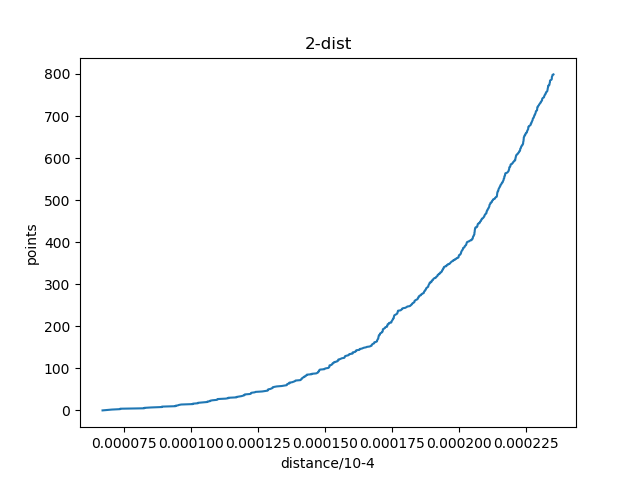python中的画图坐标问题1.鼠标点在图上就可以显示该点的横纵坐标
2.从图的中间某位置画一条竖线，将该曲线分成两部分，并能求出这条直线与曲线的交点坐标

2个回答

# -*- coding: utf-8 -*-

#任意两条曲线的交点坐标.py
import matplotlib.pyplot as plt
import numpy as np

x1 =np.linspace(-6*np.pi, 6*np.pi, 256,endpoint=True)
y2=np.sin(x1 )
y1=0.05*x1-.005
fig,ax=plt.subplots(1,1,figsize=(15,8))
plt.plot(x1,y1)#,marker='o'
plt.plot(x1,y2)#,marker='o'

data = y1-y2
doublediff =np.sign( np.diff(np.sign(np.diff(data))))
#print(doublediff)
peak_locations = np.where(doublediff == -1)
#print(peak_locations)
doublediff2 = np.sign(np.diff(np.sign(np.diff(-1*data))))
trough_locations = np.where(doublediff2 == -1)
#print(trough_locations)

ax.plot(x1[peak_locations], y2[peak_locations] , '^', markersize=10, color='m')
ax.plot(x1[trough_locations],
y2[trough_locations] ,
'^', markersize=10, color='m')

ax.set_title('title test',fontsize=12,color='r')
plt.show()

singl=np.diff(np.sign((data)))
up_locations=np.where(singl==2)
down_locations=np.where(singl==-2)

fig,ax=plt.subplots(1,1,figsize=(15,8))
plt.plot(x1,y1)#,marker='o'
plt.plot(x1,y2)#,marker='o'
ax.plot(x1[up_locations], y2[up_locations] , 'v', markersize=10, color='tab:green')
ax.plot(x1[down_locations],
y2[down_locations] ,
'^', markersize=10, color='m')
x = x1[up_locations]#np.arange(0, 6)
y = y1[up_locations]#x * x

for xy in zip(x, y):
plt.annotate("(%s,%s)" % xy, xy=xy, xytext=(-20, 10), textcoords='offset points',color='tab:green')
x1 = x1[down_locations]#np.arange(0, 6)
y1 = y1[down_locations]#x * x

for x1y1 in zip(x1, y1):
plt.annotate("(%s,%s)" % x1y1, xy=x1y1, xytext=(-20, -10), textcoords='offset points',color='tab:red')
#plt.plot(x1[:255],singl)
plt.title(' 任意两条曲线的交点坐标 ')
plt.show()Python 如何修改坐标轴单位？

python画图时，怎么设置坐标轴的单位长度？

python3.7 读取csv文件某一行用plt画图坐标轴乱，不按数字大小排列怎么解决？

python绘图，如何让坐标轴不自动显示加上一个数

python绘图，如何让坐标轴不自动显示加上一个数，如图箭头部分，如何能像图2那样显示。 ![图片说明](https://img-ask.csdn.net/upload/202004/07/1586260694_217635.png) 已解决，步骤如下： ``` import matplotlib.ticker as ticker ax=plt.gca() ax.xaxis.set_major_formatter(ticker.FormatStrFormatter('%.3f')) ax.yaxis.set_major_formatter(ticker.FormatStrFormatter('%.3f')) ```

python 散点图绘制坐标轴异常

python用matplotlib画图时如何使坐标轴的数字显示为千分位的形式如100,000,000

python用matplotlib画图时如何使坐标轴的数字显示为千分位的形式如100,000,000

python 画图plot画图时数据太多，图看起来不好看。请问怎么拉长X轴，或者增加每个点之间的间隔

matplotlib画图时坐标轴范围和刻度无法正常设置，未报错

import csv import warnings warnings.simplefilter(action='ignore', category=FutureWarning) from datetime import datetime from matplotlib import pyplot as plt file_path=r'C:\Users\YSRD-B-0281\Desktop\forth.csv' with open(file_path) as f: reader=csv.reader(f) header_row=next(reader) dates,ohs=[],[] for row in reader: current_date=datetime.strptime(row,"%Y/%m/%d %H:%M") dates.append(current_date) oh=float(row) ohs.append(row) fig=plt.figure(dpi=128,figsize=(10,6)) plt.plot(dates,ohs,c='brown',alpha=0.5) plt.title("E4",fontsize=24) plt.ylim((0,120)) plt.yticks([0,20,40,60,80,100]) plt.show() plt.close()![图片说明](https://img-ask.csdn.net/upload/201907/25/1564039180_988567.png)

ply作图坐标轴刻度问题

python在绘制图像时候，x轴的坐标未能显示

python在绘制图像时候，x轴的坐标未能显示。 显示的图片如下： ![图片说明](https://img-ask.csdn.net/upload/202004/15/1586948508_773451.png) 代码如下（本人用的是 jupyter notebook）： ``` import urllib.request import json import pygal import math from itertools import groupby # json_url = "https://raw.githubusercontent.com/muxuezi/btc/master/btc_close_2017.json" # req = urllib.request.urlopen(json_url) # json = req.read() # #将数据写入文件 # with open("E:/Jupyter/json收盘数据.json","wb") as f: # f.write(json) filename = "E:/Jupyter/json收盘数据.json" with open(filename) as f: btc_data = json.load(f) # #打印每一天的信息(获取数据过程) # for btc_dict in btc_data: # data = btc_dict["date"] # month = int(btc_dict["month"]) # week = int(btc_dict["week"]) # weekday = btc_dict["weekday"] # close = int(float(btc_dict["close"])) # print("{} is month {} week {},{},the close price is {}RMB".format(data,month,weekday,weekday,close)) #绘制收盘价折线图 dates = [] month = [] weeks = [] weekdays = [] close = [] #每一天信息 for btc_dict in btc_data: dates.append(btc_dict["date"]) month.append(int(btc_dict["month"])) weeks.append(int(btc_dict["week"])) weekdays.append(btc_dict["weekday"]) close.append(int(float(btc_dict["close"]))) #绘制收盘价对数变换价折线图 line_chart = pygal.Line(x_label_rotation = 20,show_minor_x_labels = True) line_chart.title = "收盘价" line_chart.x_label = dates N = 20 #x轴每隔20天显示一次 line_chart.x_labels_major = dates[::N] close_log = [math.log10(_) for _ in close] line_chart.add("收盘价",close_log) line_chart.render_to_file("收盘对数变换价折现图.svg") ```

python的pandas模块画图时中文显示不完全

python，读取csv文件，并画图，日期为横轴

C++(数据结构与算法)78:---分而治之

springboot+jwt实现token登陆权限认证

win10暴力查看wifi密码

MySQL数据库面试题（2020最新版）

!大部分程序员只会写3年代码

2020阿里全球数学大赛：3万名高手、4道题、2天2夜未交卷

HashMap底层实现原理，红黑树，B+树，B树的结构原理 Spring的AOP和IOC是什么？它们常见的使用场景有哪些？Spring事务，事务的属性，传播行为，数据库隔离级别 Spring和SpringMVC，MyBatis以及SpringBoot的注解分别有哪些？SpringMVC的工作原理，SpringBoot框架的优点，MyBatis框架的优点 SpringCould组件有哪些，他们...

Python爬虫，高清美图我全都要（彼岸桌面壁纸）

Java岗开发3年，公司临时抽查算法，离职后这几题我记一辈子